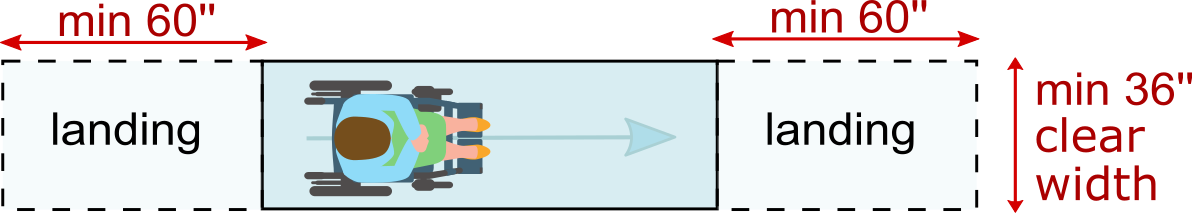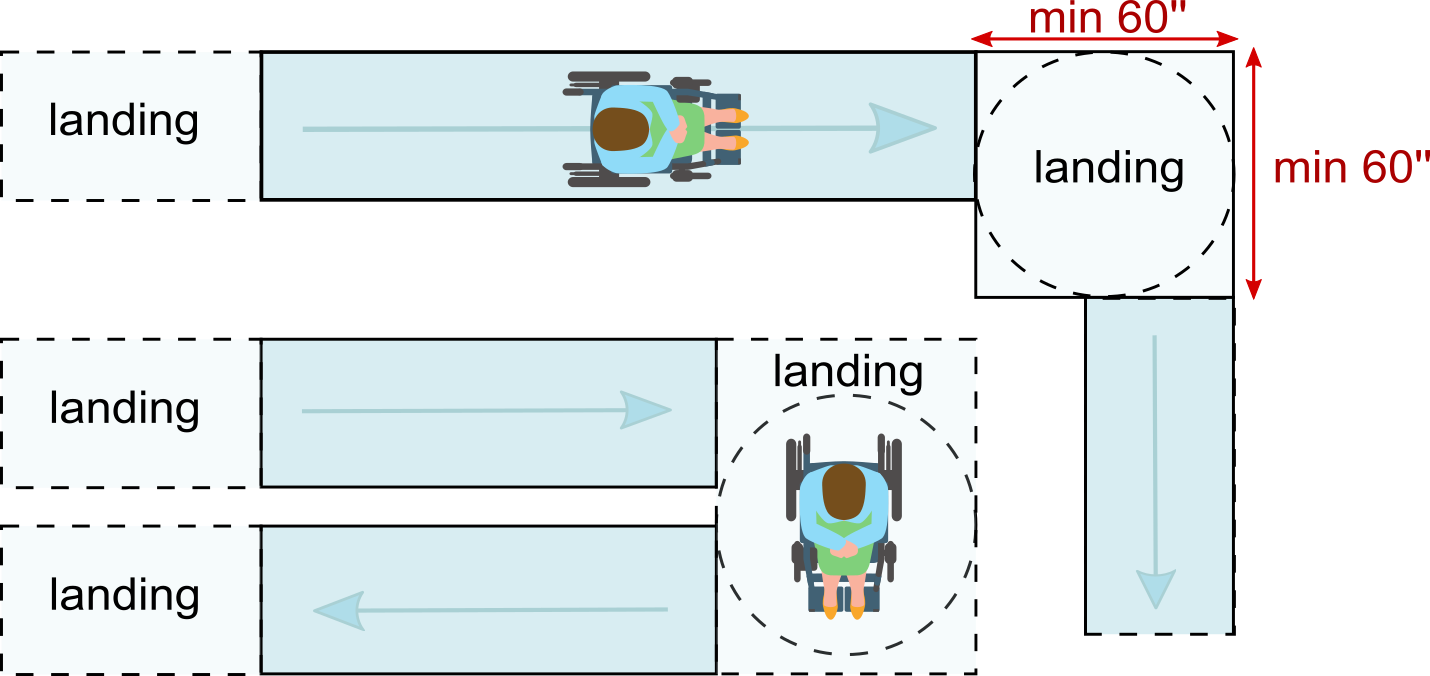# Ramp Calculator

Created by Hanna Pamuła, PhD candidate
Reviewed by Bogna Szyk and Jack Bowater
Last updated: Apr 12, 2022

If you're struggling with getting your pushchair🚼 or wheelchair♿ up some steps, we've prepared an excellent tool for you - a ramp calculator. Not only can you check the appropriate ramp slope, but we'll give you hints about ramp construction as a whole as well. No need to worry about things like ramp size, landing size, or handrails! If you'd like to know how to build a ramp that complies with ADA ramp requirements, we have included the tips. Also, we recommend reading the detailed ADA ramp slope paragraph below. Are you ready?🔨

## How do you calculate the slope of a ramp?

Before we show you how to calculate the slope of a ramp, we first need to explain slope unit convention. You can express slope in many ways, but let's focus on the three most popular forms:1. Rise to run ratio

This is the ratio of one part rise to the appropriate part of the run. It can be expressed in a few different notations: 1:8, 1/8, 1 in 8, e.g. for example, with a 1:12 ratio, for each unit of vertical rise, you need to have 12 units of horizontal run, so 1 inch of rise means that 12 inches (1 foot) of horizontal space is needed. Common slopes are 1:12, 1:20, 1:8.

slope[ratio] = rise : run

You can find the missing term of any ratio with our ratios calculator!

1. Angle

It's the angle between ramp and run (or, in other words, angle of inclination to the horizontal). This angle is commonly expressed in degrees, though other angle units are possible. To calculate the slope's angle, use the inverse tangent:

slope[angle] = arctan(rise/run)

1. Percentages

Giving the grade (slope) as a percentage is the most common way of describing slopes in the US and in Europe. Be careful - a 100% slope is not an equivalent to 90 degrees, but to 45 degrees. It's calculated from the tangent of the inclination angle:

slope[%] = 100% * rise/run = 100% * tan(angle)Slope as fraction, percentage and in degrees

After that intro on slope units, let's come back to the heart of the matter: how do you calculate the slope of a ramp?Well, that depends what information you have - if you know the rise and run (the easiest case) then you can use the formulas mentioned above. However, you might know the rise and the hypotenuse of the right triangle the ramp forms. In this case, you can calculate the run directly from Pythagorean formula:

run2 + rise2 = hypotenuse2

run = √(hypotenuse2 - rise2)

In this wheelchair ramp slope calculator, ramp length is the hypotenuse, but for stairs it will be the stringer length, and for roofing - the rafter length.

Alternatively, you can find the slope using inverse functions:

• inverse sin, if you know the rise and the hypotenuse:

slope[%] = arcsin(rise/hypotenuse)

• inverse cosine, if you know the run and the hypotenuse:

slope[%] = arccos(rise/hypotenuse)

## ADA ramp slopes

The (Americans with Disabilities Act) set out some minimum requirements for new public facilities. As well as including many different accessibility standards, it contains guidelines for ramp construction. In this paragraph, we'll take a closer look at ADA ramp slopes.

According to the ADA standards, the least steep slope shall be used for any ramp. Apart from that information, what exactly does the guideline say?

• 1:12 is the maximum slope of a ramp for new constructions• 1:16 is the comfortable slope• 1:20 is the minimum slope - below that value it's not considered a ramp (thus e.g., handrails are not needed)In each case, the maximum rise allowed is 30 inches (760 mm). These three ADA ramp slope values are preset in our ramp calculator.

If existing sites, buildings, and facilities have space limitations, steeper ADA ramp slopes may be permitted. However, stricter restrictions on the maximum rise apply:

Slope

Maximum rise

inches

millimeters

1:8 to <1:10

3 inches

75 mm

1:10 to <1:12

6 inches

150 mm

Remember that the ADA prohibits slopes steeper than 1:8.

## Other ADA ramp requirements

When constructing a ramp, be it for your home, public space or temporary use, you need to think about a few things other than a ramp slope and rise:

1. Clear Width

The minimum clear width of a ramp is 36 in (91.5 cm). Don't forget to take into account the mounting of handrails when designing a ramp.

2. Landings

Every ramp should have level landings at the top and bottom of each section - especially if your ramp has multiple sections (turns). The following landing features are essential:

• Minimum landing length is 60 in (1525 mm), and the landing's width should be at least as wide as the ramp's run.• You need to provide enough space for maneuvers: if the ramp construction changes direction (a 90 degree turn), then the minimum landing size is 60"x60":Don't forget to add even more space for switchback ramps.

3. Handrails

Every time a ramp has:

• a rise greater than 6 in (15 cm) or

• a run greater than 72 in (183 cm),

then the handrails are needed on both sides to comply with ADA standards. You will find more details in paragraph 4.8.5 of the

4. Other important factors are:

• the cross slope (camber) of the ramps cannot exceed 1:50,
• the ramp should be made out of appropriate material to prevent sliding and slipping,
• edge protection and handrail extensions,
• taking into account weather conditions if it's an outdoor ramp (proper drainage).

## Wheelchair ramp slope calculator. Calculate🖩, design📝, and DIY🔨

If you're wondering how to build a ramp, our ramp calculator will be a great help in its design and construction. Use it as either an ADA ramp slope calculator to meet the government's standards, or as a tool to calculate the ramp explicitly tailored to your needs.

Now, how to use this wheelchair ramp slope calculator?

1. Choose the ramp's application. Let's assume that we want to comply with the ADA ramp requirements, and we'd like to make a comfortable ramp. Pick the 1:16 option from a drop-down list.

2. Select the ramp type. You can choose from a straight ramp, a ramp with one turn, or a ramp with a switchback. Select the latter for our example.

3. Input the vertical rise. In our case, we need to overcome five 7-inch steps. Enter 35 into the rise box (or simply 5*7).

4. And that's it - our ADA ramp slope calculator will show you your results:

• slope: 3.58° (6.25 %)

• run: 46.67 ft

• ramp length: 46.67 ft

• useful info for two ramp runs construction:

For two ramps of equal length, each should be 23.38 ft long. Of course, you can split it differently, depending on the space available. Just remember that for such slope, the maximum distance between landing sites is 40 ft.

Additionally, you get some tips about landing size and ramp width.

That wasn't so hard, was it?

Moreover, our tool is even more versatile than that, as you don't need to choose from the preset applications. For example, if you want a ramp with a specific angle, just enter that value into the proper box (e.g., 5°), fill in the rise field, and the run and ramp length will be calculated for you. Also, you can hit the Advanced mode button to show the slope in ratio form, and then you can input the slope ratio value (e.g., if you need a 1:8 ratio for old buildings).

We hope that our ramp calculator will be beneficial to you. Rate this calculator and tell us what you think about it and if it can be improved it in any way.

## FAQ

### How to calculate the angle of a ramp?

To calculate the angle of a ramp:

1. Picture the ramp as a right triangle. Lets call the vertical height 'b', the horizontal distance 'a', and the ramp path 'c'. You will need to find at least two of the three values.
2. If you have 'a' and 'b', divide the arctangent of 'b' by 'a'.
3. If you have 'c' and either 'a' or 'b', you can find the arccosine (a/c) or arcsine (b/c), respectively. We recommend using an online calculator to solve this step.

### What is a 100% slope?

A 100% slope is one that is 45 degrees above the horizontal. It also has the same horizontal length (run) and the vertical length (rise). The following formula explains it:

• Slope percentage = 100% × tangent (slope angle)

Inputting our values and rearranging for slope angle gives:

• slope angle = arctan(1)
• slope angle = 45°

### What is the minimum and maximum slope allowed?

The range of allowed slope for a wheelchair ramp will depend on your local regulation. One of the most famous ones is the ADA Ramp Requirement code. It establishes the following limits:

• Minimum slope: 1:20, meaning you will have 20 units of horizontal length (run) by 1 unit of vertical length (rise).
• Maximum slope: 1:12, meaning you will have 12 units of horizontal length (run) by 1 unit of vertical length (rise).

### How to build a ramp?

To build a ramp for a wheelchair:

1. Keep at least a width of 91.5 cm (36 inches).
2. Measure the vertical length you want to cover. Consider the slope ratio requirement your local regulations demand. For example, ADA requires a minimum slope of 1:20 and a maximum of 1:12.
3. Ensure the landing area is at least 1.525 meters (60 inches) long.
4. For further building construction, check a dedicated wheelchair ramp slope calculator.
Hanna Pamuła, PhD candidate
Application
Unassisted user, ADA max slope (1:12)Ramp type
straight
Rise
inRamp
Slope
deg
%
Run
ft
Ramp length
ft
People also viewed…

### Bolt torque

The bolt torque calculator helps you make sure you tighten your bolts correctly.

### Lost socks

Socks Loss Index estimates the chance of losing a sock in the laundry.

### Roof shingle

With this roof shingle calculator's help, you will be able to quickly estimate how many bundles of shingles you need for your roof.

### Steps to calories

Steps to calories calculator helps you to estimate the total amount to calories burned while walking.